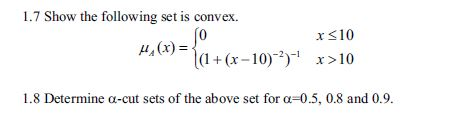# 1.7 Show the following set is convexx s10μ^ (x) =t(1 + (x-10)-がx>101.8 Determine α-cut sets of the above set for α-05, 0.8 and 0.9.

Question
135 views

the book is "First Course on Fuzzy Theory and Applications"help_outlineImage Transcriptionclose1.7 Show the following set is convex x s10 μ^ (x) =t(1 + (x-10)-が x>10 1.8 Determine α-cut sets of the above set for α-05, 0.8 and 0.9. fullscreen
check_circle

Step 1

To prove that the given fuzzy set is convex and to determine the α-cut sets for the given values of α.

Step 2

Membership function of the fuzzy set A

Step 3

Definition of a convex...

### Want to see the full answer?

See Solution

#### Want to see this answer and more?

Solutions are written by subject experts who are available 24/7. Questions are typically answered within 1 hour.*

See Solution
*Response times may vary by subject and question.
Tagged in

### Math